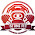Thursday, June 25, 2015

39 118 | The Death of Pumpkinhead at Age 39 on June 9, 2015 (Making Madison Smile)The latest Freemason Hip-Hop Sacrifice. Freemason = 96 June 6 is written 6/9 or 9/6
Pumpkinhead was born Robert Diaz.
• Pumpkinhead = 7+3+4+7+2+9+5+8+5+1+4 = 55
• Robert = 9+6+2+5+9+2 = 33
• Diaz = 4+9+1+8 = 22
• Robert Diaz = 55
His death at 39 connects to Hip-Hop music.
• Hip 8+9+16 = 33 (Rhyme = 33)(Orange = 33)(Orange has no rhyme)
• Hop = 8+15+16 = 39
• NY = 14+15 = 39
• New York = 5+5+5+7+6+9+2 = 39
• Empire = 5+4+7+9+9+5 = 39
• 7/26/1788 = 7+2+6+1+7+8+8 = 39
• UN is 39 Floors in NY
• The New York Times = 93 (9/18/1851 = 9+1+8+1+8+5+1 = 33)
• One World Trade Center = 93
• '93 WTC Bombing
• Flight 93 of 33 Passengers
• Flight 11 Hit Floors 93 through 99
• Madison = 39
• Madison made 39 proposal that became the Bill of Rights (6/8/1789 = 6+8+1+8+7+9 = 39)
• Madison Authored Federalist Paper #39 on 1/18/1789Dynamic Song?  Dynamic = 4+7+5+1+4+9+3 = 33
Notice that the reported time of death is 5:40...
• Thelema worships the number 93, the reverse of 39
• Thelema is about 'Do What Thou Wilt' and 'Love'
• Love = 12+15+22+5 = 54
• Sun = 19+21+14 = 54
Notice the time of the tweet below...  Do you think "Marco Polo" knows a thing or two?

1.